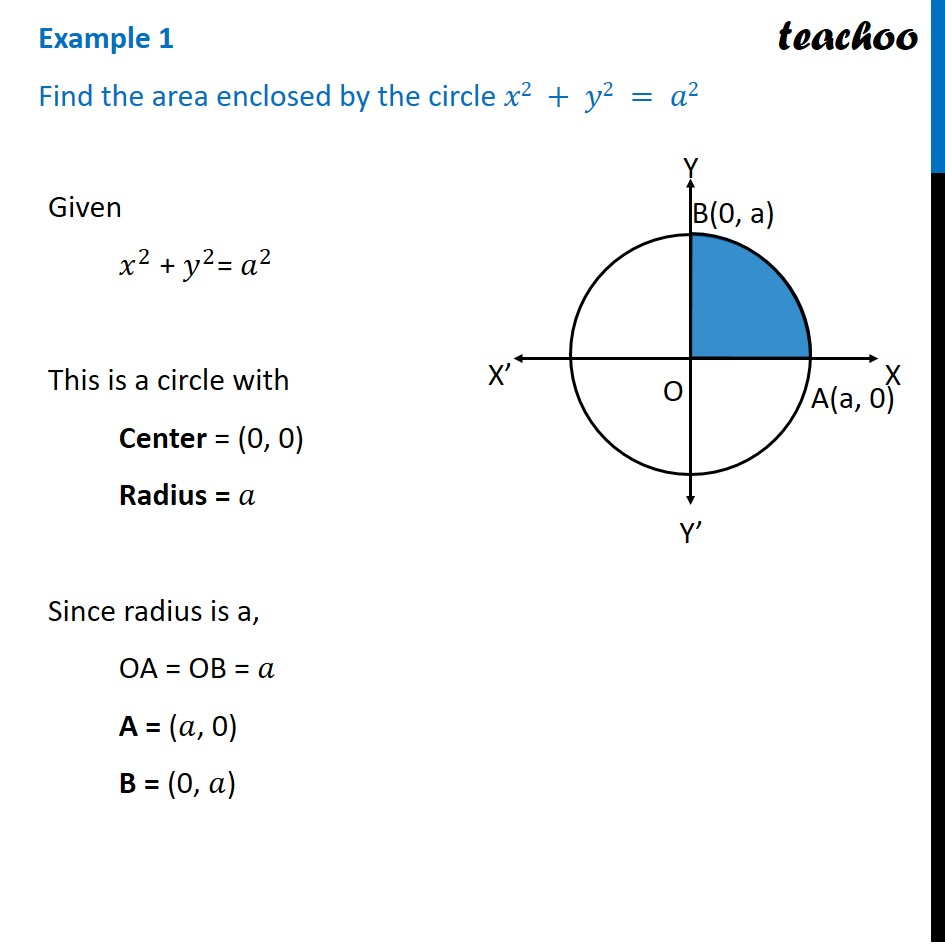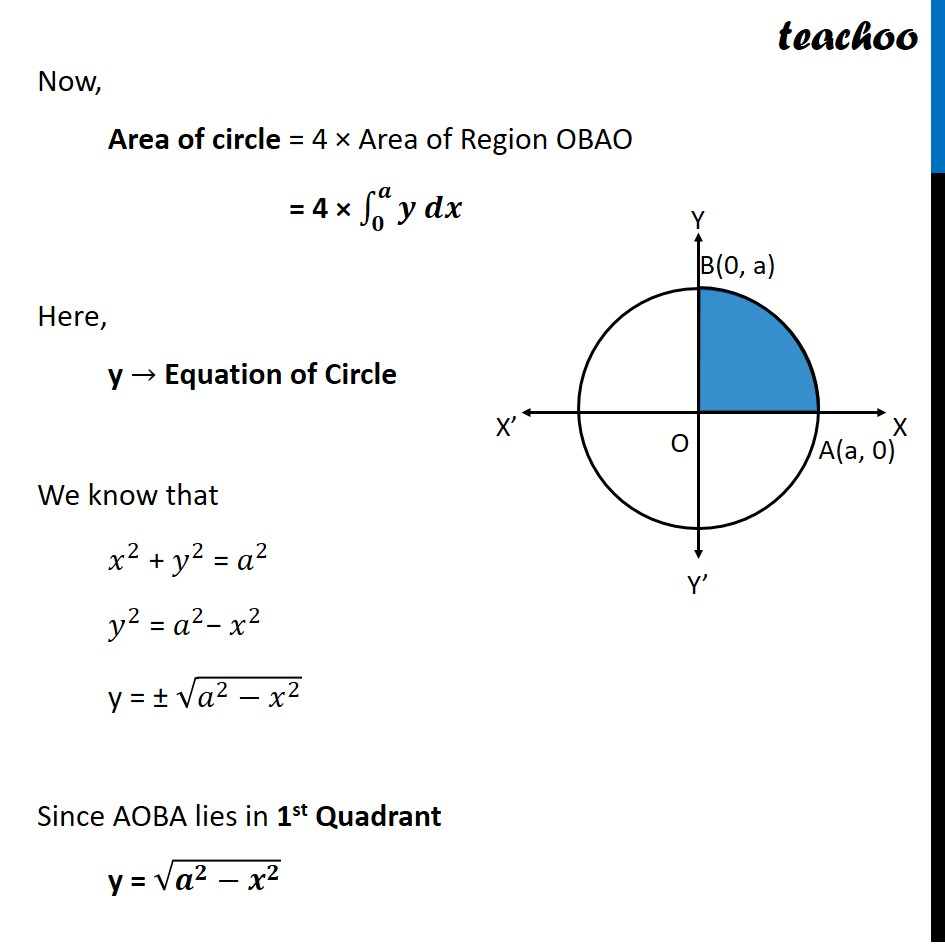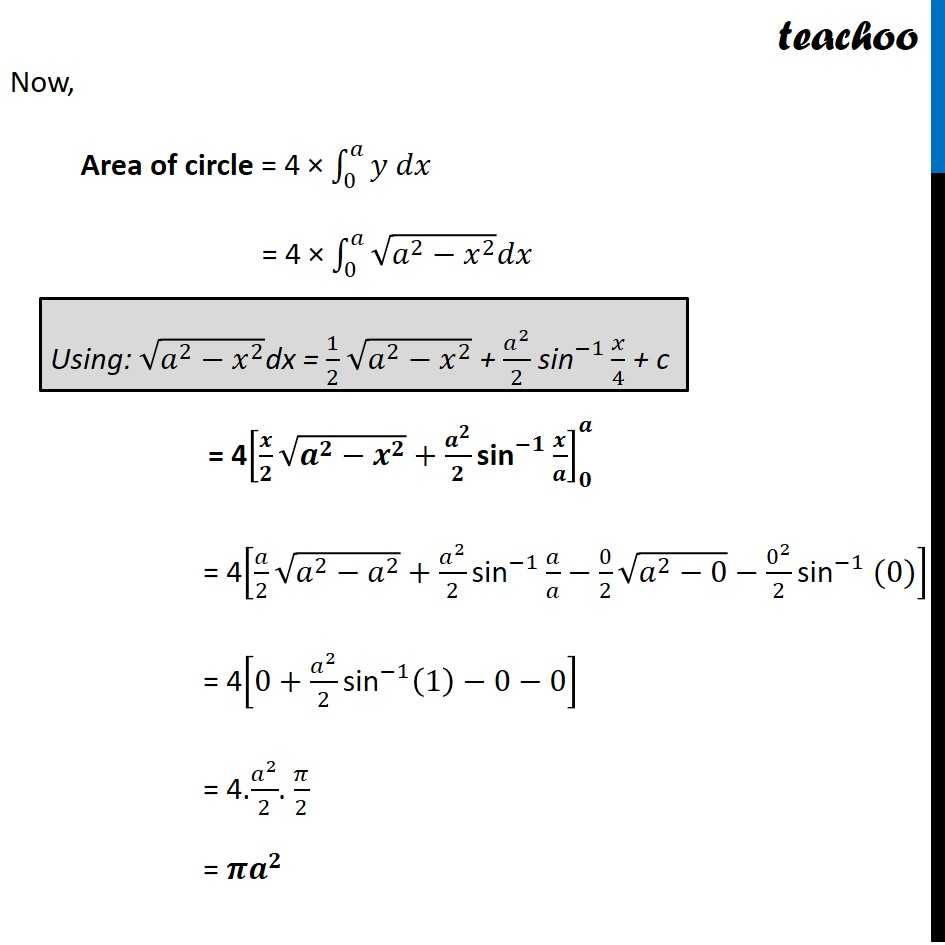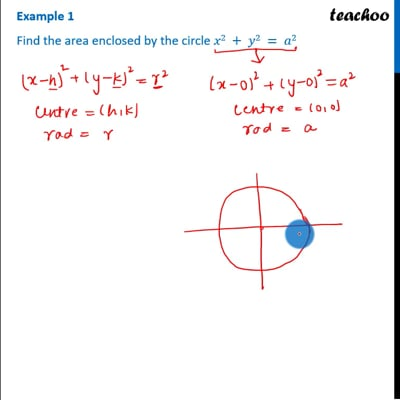Examples

Chapter 8 Class 12 Application of Integrals
Serial order wiseThis video is only available for Teachoo black users

Solve all your doubts with Teachoo Black (new monthly pack available now!)

### Transcript

Example 1 Find the area enclosed by the circle 𝑥2 + 𝑦2 = 𝑎2Given 𝑥^2 + 𝑦^2= 𝑎^2 This is a circle with Center = (0, 0) Radius = 𝑎 Since radius is a, OA = OB = 𝑎 A = (𝑎, 0) B = (0, 𝑎) Now, Area of circle = 4 × Area of Region OBAO = 4 × ∫1_𝟎^𝒂▒〖𝒚 𝒅𝒙〗 Here, y → Equation of Circle We know that 𝑥^2 + 𝑦^2 = 𝑎^2 𝑦^2 = 𝑎^2− 𝑥^2 y = ± √(𝑎^2−𝑥^2 ) Since AOBA lies in 1st Quadrant y = √(𝒂^𝟐−𝒙^𝟐 ) Now, Area of circle = 4 × ∫1_0^𝑎▒〖𝑦 𝑑𝑥〗 = 4 × ∫1_0^𝑎▒〖√(𝑎^2−𝑥^2 ) 𝑑𝑥〗 = 4[𝒙/𝟐 √(𝒂^𝟐−𝒙^𝟐 )+𝒂^𝟐/𝟐 〖"sin" 〗^(−𝟏) 𝒙/𝒂]_𝟎^𝒂 = 4[𝑎/2 √(𝑎^2−𝑎^2 )+𝑎^2/2 〖"sin" 〗^(−1) 𝑎/𝑎−0/2 √(𝑎^2−0)−0^2/2 〖"sin" 〗^(−1) (0)] = 4[0+𝑎^2/2 〖"sin" 〗^(−1) (1)−0−0] = 4.𝑎^2/2. 𝜋/2 = 𝝅𝒂^𝟐 Using: √(𝑎^2−𝑥^2 )dx = 1/2 √(𝑎^2−𝑥^2 ) + 𝑎^2/2 〖"sin" 〗^(−1) 𝑥/4 + c